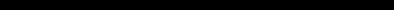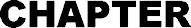# The ZX80 Operating ManualFINDING THE ANSWERS Up to now you've just been getting used to the feel of the computer. Now we'll actually use the computer to do a few sums. THE LET STATEMENT Nearly all arithmetic operations are done by using the LET statement. It is of the form LET variable = expression. In this case the variable (which can have any name you assign to it) is what you want to find out. The expression describes how you want to define the variable. For instance  LET A        = 2+4 variable     expression means "add 2 to 4 and set A equal to the result (6)". You can use other variables: LET A = 2 + B or LET A = B + C One condition: the computer must already know what the values of B and C are before it comes to the LET statement. The equal sign (=) isn't used in quite the same way it is in ordinary arithmetic or algebra. For instance: LET J = J +1 Obviously J isn't equal to J + 1. What the LET statement means is: "Take the existing value of J, add one to it and then set J to this value." The + sign is called an operator and defines the operation you wish to be performed. The other arithmetic operators are: -     (minus) *     (multiply - there is no x sign to avoid confusion with letter x) /     (divide) **     (raise to the power) For the moment we'll leave / and ** out of it. Here is a program for multiplying two numbers together 10 PRINT "MULTIPLICATION" 20 PRINT "ENTER FIRST NUMBER" 30 INPUT A 40 PRINT "ENTER SECOND NUMBER" 50 INPUT B 60 LET C==A * B 70 PRINT "THE ANSWER IS", C Notice line 70. It's a PRINT statement with a string between inverted commas, but it's got a C added to it. This form allows you to economize on PRINT statements. Enter the program and try running it, using two quite small numbers  such as 17 and 25. The computer will come up with the answer: THE ANSWER IS 425 Run the program a few more times, increasing the size of the input variables. At about 255 * 129 or just a bit larger you will get another error message  6/60. The 6 is the code for arithmetic overflow because the answer is larger than 32767, which is the largest number the computer can hold as an integer variable. Now try editing the program so that it does addition instead - just change the * to a + in line 60. You can also try subtraction and check that you get negative numbers when A is less than B. All fairly straightforward so far. Now let's try division. Alter line 60 to 60 LET C = A/B Run the program for A (first number) = 24 B (second number) = 12 The computer will come up with: THE ANSWER IS 2 Run the program again, this time for A=18 and B=12 THE ANSWER IS 1 ??!! Shouldn't that be 1.5? Well, yes, but the ZX-80 uses integer arithmetic  only whole numbers can be expressed. What the computer does is to do a division normally and the truncate the result towards ZERO. As examples: ANSWER -2.58        would become-2 -0.01        would become 0 -212.1      would become -212 -5941.98  would become -5941 This means that you may have to be careful when you use division  it's always fairly accurate when you're dividing a large number by a small number, but may be less accurate when the two numbers are closer together in size. Luckily there are ways of getting round this problem. For instance, here is a program which will give quotients to 3 decimal places using integer arithmetic only. 10 PRINT DIVISION PROGRAM 20 PRINT DIVIDEND = ? 30 INPUT X 40 PRINT DIVISOR = ? 50 INPUT Y 60 LET Z = X/Y      Divides X by Y 70 LET R1 = X-Z*Y      Calculates Remainder 80 LET D1 = 10*R1/Y    Divides 10*remainder by Y to give first decimal 90 LET R2 = 10*R1-D1*Y     Calculates 2nd remainder 100 LET D2 = 10*R2-D2*Y     Divides to give 2nd decimal place 110 LET R3 = 10*R2-D2*Y Calculates 3rd remainder 120 LET D3 = 10*R3/Y     Last decimal place 130 PRINT THE ANSWER IS ;Z;.;D1;D2;D3 This program does a long division in exactly the same way as we would do it on paper. Try running it! Notice the PRINT list in line 130  it contains several literal strings and variables separated by semicolons. The semicolons tell the ZX-80 that each thing to be printed must be printed immediately after the preceding item, without spaces between them. Also notice the LET statements from line 70 on. There's no fiddle  you can use more than one operator per statement. There is a snag, though. Operations are not necessarily carried out in order from left-to-right. The order in which they are carried out depends on what sort of operations are present in the statement. Here is a list of priorities for arithmetic operations: First     A**B     (A to the power B) Second     A    (Negation, or multiplication by-1) Third     A*B Fourth     A/B Fifth     A+B or A-B Now for some experiments 10 REM TEST PROGRAM (REM is a keyword  key Y) 20 PRINT "ENTER A" 30 INPUT A 40 PRINT "ENTER B" 50 INPUT B 60 PRINT "ENTER C" 70 INPUT C 80 LET Z = A + B * C 90 PRINT Z Try running this with A=1, B=2, C=3 but before you start, use the priority rules to predict what answer the ZX-80 will give: 7 or 9? O.K.  run it now. The answer was 7. First the computer multiplied 2 by 3 to get 6, then it added 1 to get the final answer. Try a few other combinations by editing statement 80 - like A*B/C A/B*C     (watch out for truncation!) A**B     (A to the power B  try using small numbers with C = 0) -A**B A*-B Here's a golden rule: when in doubt either use more than one LET statement and build up that way, or use brackets. Brackets make life much easier when it comes to complex arithmetic operations. Take example Z = A/B*C The normal sequence would be for the computer to evaluate B times C and then divide A by the result. If we put brackets round A/B thus: 80 LET Z = (A/B)*C then the computer will carry out the operation within the brackets first, even though it has a lower priority. A more subtle example is: Z=A*(B/C) and Z = A*B/C At first sight you would think that these would give the same answer. Well, they do  sometimes! Try them both using A == 100, B = 25, C = 5 (the answer is 500). Now try it both ways using A = 100, B = 3, C = 5 (the answer should be 60). What happened when you used the brackets? Think about it. The phantom truncator has struck again! The computer evaluated 3/5 first and truncated it to ZERO - then it multiplied 100 by ZERO and naturally got ZERO as a result. All this emphasizes that you have to be very much on the alert when you use integer arithmetic for multiplication and division. Multiplications can cause arithmetic overflow problems (which will cause the program to stop), whilst small numbers used in division may give rise to funny answers which don't give error codes. If you think that you're going to run into truncation then do multiplications first  on the other hand it may be better to do divisions first to avoid overflow. That's probably enough arithmetic for now. Let's move on to more interesting things.